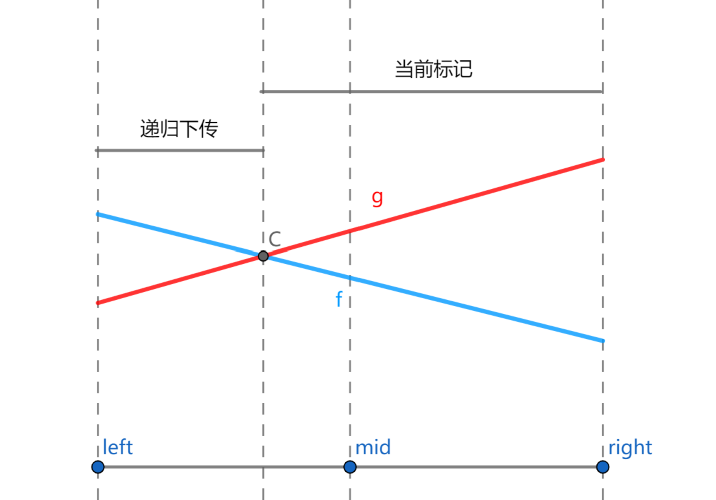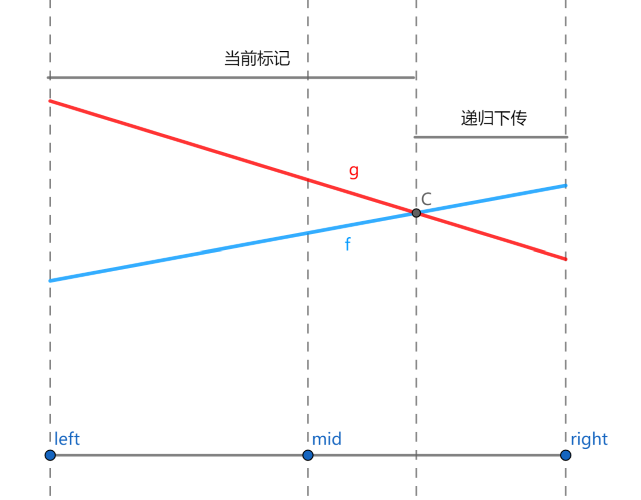• ChungZH's blog
• ## 李超线段树笔记

• @ 2022-8-20 10:23:46

## 线段树之标记永久化

1. 比如我们先设置后加，并且令设置的区间比加的区间大，因此加的 tag 在下方，设置的 tag 在上方。
2. 根据前面的方法，我们会从下往上取 tag，也就是先加法，再设置。
3. 这时我们发现，由于上层是一个设置操作，下面的所有答案最终都变成了设置的那个数字，下层操作就失效了。显然有问题。

int up(int p) { tree[p].mx = max(tree[p<<1].mx, tree[p<<1|1].mx)+tree[p].tag; }
int query(int p, int l, int r, int x, int y) {
if (l >= x && r <= y) return tree[p].mx;
int mid = (l + r) / 2, ans = -INF;
if (x <= mid) ans = max(ans, query(p << 1, l, mid, x, y));
if (y > mid) ans = max(ans, query(p << 1 | 1, mid + 1, r, x, y));
return ans+tree[p].tag;
}
void update(int cur, int l, int r, int x, int y, int c) {
if (l >= x && r <= y) {
tree[cur].tag += c;
tree[cur].mx += c;
return;
}
int mid = (l + r) / 2;
if (x <= mid) update(cur << 1, l, mid, x, y, c);
if (y > mid) update(cur << 1 | 1, mid + 1, r, x, y, c);
up(cur);
}


## 李超线段树

1. 在平面上加入一条线段。记第 $i$ 条被插入的线段的标号为 $i$，该线段的两个端点分别为 $(x_0,y_0)$$(x_1,y_1)$
2. 给定一个数 $k$，询问与直线 $x = k$ 相交的线段中，交点纵坐标最大的线段的编号（若有多条线段与查询直线的交点纵坐标都是最大的，则输出编号最小的线段）。特别地，若不存在线段与给定直线相交，输出 $0$

• 加入一个一次函数，定义域为 $[l,r]$
• 给定 $k$，求定义域包含 $k$ 的所有一次函数中，在 $x=k$ 处取值最大的那个，如果有多个函数取值相同，选编号最小的。1. 如果新线段 $f$ 更优，则将 $f$$g$ 交换。转化成了第 2 种情况。
2. 对于中点处 $f$ 不如 $g$ 优的情况：
• 若在左端点处 $f$ 更优，那么 $f$$g$ 必然在左半区间中产生了交点，$f$ 只有在左区间才可能优于 $g$，递归到左儿子中进行下传；
• 若在右端点处 $f$ 更优，那么 $f$$g$ 必然在右半区间中产生了交点，$f$ 只有在右区间才可能优于 $g$，递归到右儿子中进行下传；
• 若在左、右端点处 $g$ 都更优，那么 $f$ 不可能成为答案，不需要继续下传。

RECORD

#include <bits/stdc++.h>

using namespace std;
const int N = 1000005;
const int MOD1 = 39989;
const int MOD2 = 1000000000;
const double EPS = 1e-12;
struct line {
double k, b;
int l, r;
int id;
} tree[N << 2];
int n;
double calc(line a, int x) {  // 计算纵坐标
return x * a.k + a.b;
}
// 注意 cmp 写法。不要写成 a-b < EPS。
int cmp(double a, double b) {
if (a - b > EPS) return 1;
if (b - a > EPS) return -1;
return 0;
}
void build(int rt, int l, int r) {
tree[rt] = {0, 0, 1, 40000, 0};
if (l == r) return;
int m = (l + r) >> 1;
build(rt << 1, l, m);
build(rt << 1 | 1, m + 1, r);
}
void modify(int root, int l, int r, line k) {
if (l >= k.l && r <= k.r) { // 对线段完全覆盖到的区间进行修改
// g: tree[root]; f: k.
int m = (l + r) >> 1;
if (cmp(calc(tree[root], m), calc(k, m)) == -1) swap(tree[root], k);

int cl = cmp(calc(k, l), calc(tree[root], l)),
cr = cmp(calc(k, r), calc(tree[root], r));
// 3. 若在左右端点处 g 都更优，那么 f 不可能成为答案，不需要继续下传。
if (cl == -1 && cr == -1) return;
// 1. & 2. 注意题目要求下标尽量小，下标小的线段即使不更优，如果值相等，也可以下传。
if (cl == 1 || (cl == 0 && k.id < tree[root].id)) modify(root << 1, l, m, k);
if (cr == 1 || (cr == 0 && k.id < tree[root].id)) modify(root << 1 | 1, m + 1, r, k);
} else {
int m = (l + r) >> 1;
if (k.l <= m) modify(root << 1, l, m, k);
if (k.r > m) modify(root << 1 | 1, m + 1, r, k);
}
}
pair<double, int> pmax(pair<double, int> x,
pair<double, int> y) {  // 注意题目要求下标尽量小
if (cmp(x.first, y.first) == -1)
return y;
else if (cmp(x.first, y.first) == 1)
return x;
else
return x.second < y.second ? x : y;
}

pair<double, int> query(int root, int l, int r, int x) {
if (l == r) return {calc(tree[root], x), tree[root].id};

int m = (l + r) >> 1;
pair<double, int> ans = {calc(tree[root], x), tree[root].id};
// 注意标记永久化，取 max 比较
if (x <= m)
ans = pmax(ans, query(root << 1, l, m, x));
else
ans = pmax(ans, query(root << 1 | 1, m + 1, r, x));
return ans;
}
int main() {
ios::sync_with_stdio(false);
cin >> n;
build(1, 1, 40000);
int lastans = 0;
int segi = 0;
while (n--) {
int op;
cin >> op;
if (op == 0) {
int k;
cin >> k;
k = (k + lastans - 1 + MOD1) % MOD1 + 1;
lastans = query(1, 1, 40000, k).second;
cout << lastans << endl;
} else {
int a, b, x, y;
cin >> a >> b >> x >> y;
a = (a + lastans - 1 + MOD1) % MOD1 + 1;
b = (b + lastans - 1 + MOD2) % MOD2 + 1;
x = (x + lastans - 1 + MOD1) % MOD1 + 1;
y = (y + lastans - 1 + MOD2) % MOD2 + 1;
if (a > x) {
swap(a, x);
swap(b, y);
}
line t;
if (a == x) {  // 垂直于 y 的函数的特殊处理
t.k = 0;
t.b = max(b, y);
} else {
t.k = (double)(y - b) / (x - a);
t.b = b - t.k * a;
}
t.l = a;
t.r = x;
t.id = ++segi;
modify(1, 1, 40000, t);
}
}
return 0;
}